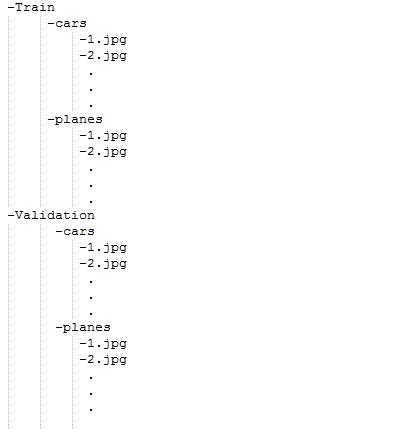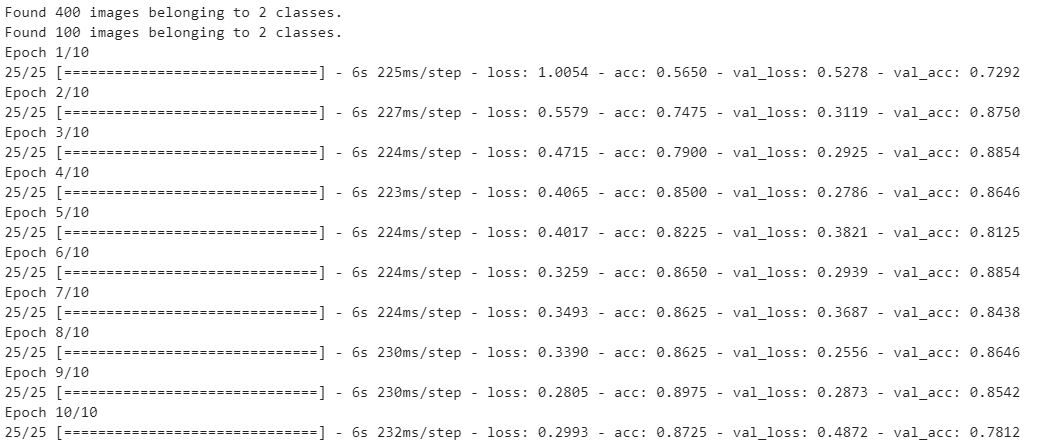Skip to content
Related Articles
Python | Image Classification using keras
• Difficulty Level : Medium
• Last Updated : 24 Apr, 2020

Prerequisite: Image Classifier using CNN

Image classification is a method to classify the images into their respective category classes using some method like :

• Training a small network from scratch
• Fine tuning the top layers of the model using VGG16

Let’s discuss how to train model from scratch and classify the data containing cars and planes.

Train Data : Train data contains the 200 images of each cars and planes i.e. total their are 400 images in the training dataset
Test Data : Test data contains 50 images of each cars and planes i.e. total their are 100 images in the test dataset

To download the complete dataset, click here.

Model Description: Before starting with the model firstly prepare the dataset and it’s arrangement. Look at the following image given below:For feeding the dataset folders the should be made and provided into this format only. So now, Let’s begins with the model:
For training the model we don’t need a large high end machine and GPU’s, we can work with CPU’s also. Firstly, in given code include following libraries:

 `# Importing all necessary libraries``from` `keras.preprocessing.image ``import` `ImageDataGenerator``from` `keras.models ``import` `Sequential``from` `keras.layers ``import` `Conv2D, MaxPooling2D``from` `keras.layers ``import` `Activation, Dropout, Flatten, Dense``from` `keras ``import` `backend as K`` ` `img_width, img_height ``=` `224``, ``224`

Every image in the dataset is of the size 224*224.

 `train_data_dir ``=` `'v_data/train'``validation_data_dir ``=` `'v_data/test'``nb_train_samples ``=``400` `nb_validation_samples ``=` `100``epochs ``=` `10``batch_size ``=` `16`

Here, the `train_data_dir` is the train dataset directory. `validation_data_dir` is the directory for validation data. `nb_train_samples` is the total number train samples. `nb_validation_samples` is the total number of validation samples.

Checking format of Image:

 `if` `K.image_data_format() ``=``=` `'channels_first'``:``    ``input_shape ``=` `(``3``, img_width, img_height)``else``:``    ``input_shape ``=` `(img_width, img_height, ``3``)`

This part is to check the data format i.e the RGB channel is coming first or last so, whatever it may be, model will check first and then input shape will be feeded accordingly.

 `model ``=` `Sequential()``model.add(Conv2D(``32``, (``2``, ``2``), input_shape``=``input_shape))``model.add(Activation(``'relu'``))``model.add(MaxPooling2D(pool_size``=``(``2``, ``2``)))`` ` `model.add(Conv2D(``32``, (``2``, ``2``)))``model.add(Activation(``'relu'``))``model.add(MaxPooling2D(pool_size``=``(``2``, ``2``)))`` ` `model.add(Conv2D(``64``, (``2``, ``2``)))``model.add(Activation(``'relu'``))``model.add(MaxPooling2D(pool_size``=``(``2``, ``2``)))`` ` `model.add(Flatten())``model.add(Dense(``64``))``model.add(Activation(``'relu'``))``model.add(Dropout(``0.5``))``model.add(Dense(``1``))``model.add(Activation(``'sigmoid'``))`

About the following terms used above:

`Conv2D` is the layer to convolve the image into multiple images
`Activation` is the activation function.
`MaxPooling2D` is used to max pool the value from the given size matrix and same is used for the next 2 layers. then, `Flatten` is used to flatten the dimensions of the image obtained after convolving it.
`Dense` is used to make this a fully connected model and is the hidden layer.
`Dropout` is used to avoid overfitting on the dataset.
`Dense` is the output layer contains only one neuron which decide to which category image belongs.

Compile Function:

 `model.``compile``(loss``=``'binary_crossentropy'``,``              ``optimizer``=``'rmsprop'``,``              ``metrics``=``[``'accuracy'``])`

Compile function is used here that involve use of loss, optimizers and metrics.here loss function used is `binary_crossentropy`, optimizer used is `rmsprop`.

Using DataGenerator:

 `train_datagen ``=` `ImageDataGenerator(``    ``rescale``=``1.` `/` `255``,``    ``shear_range``=``0.2``,``    ``zoom_range``=``0.2``,``    ``horizontal_flip``=``True``)`` ` `test_datagen ``=` `ImageDataGenerator(rescale``=``1.` `/` `255``)`` ` `train_generator ``=` `train_datagen.flow_from_directory(``    ``train_data_dir,``    ``target_size``=``(img_width, img_height),``    ``batch_size``=``batch_size,``    ``class_mode``=``'binary'``)`` ` `validation_generator ``=` `test_datagen.flow_from_directory(``    ``validation_data_dir,``    ``target_size``=``(img_width, img_height),``    ``batch_size``=``batch_size,``    ``class_mode``=``'binary'``)`` ` `model.fit_generator(``    ``train_generator,``    ``steps_per_epoch``=``nb_train_samples ``/``/` `batch_size,``    ``epochs``=``epochs,``    ``validation_data``=``validation_generator,``    ``validation_steps``=``nb_validation_samples ``/``/` `batch_size)`

Now, the part of `dataGenerator `comes into figure.In which we have used:

`ImageDataGenerator` that rescales the image, applies shear in some range, zooms the image and does horizontal flipping with the image. This ImageDataGenerator includes all possible orientation of the image.
`train_datagen.flow_from_directory` is the function that is used to prepare data from the train_dataset directory `Target_size` specifies the target size of the image.
`test_datagen.flow_from_directory` is used to prepare test data for the model and all is similar as above.
`fit_generator` is used to fit the data into the model made above, other factors used are `steps_per_epochs` tells us about the number of times the model will execute for the training data.
`epochs` tells us the number of times model will be trained in forward and backward pass.
`validation_data` is used to feed the validation/test data into the model.
`validation_steps` denotes the number of validation/test samples.

 `model.save_weights(``'model_saved.h5'``)`

At last we can also save the model.

Below is the complete implementation:

 `# importing libraries``from` `keras.preprocessing.image ``import` `ImageDataGenerator``from` `keras.models ``import` `Sequential``from` `keras.layers ``import` `Conv2D, MaxPooling2D``from` `keras.layers ``import` `Activation, Dropout, Flatten, Dense``from` `keras ``import` `backend as K`` ` ` ` `img_width, img_height ``=` `224``, ``224`` ` `train_data_dir ``=` `'v_data/train'``validation_data_dir ``=` `'v_data/test'``nb_train_samples ``=` `400` `nb_validation_samples ``=` `100``epochs ``=` `10``batch_size ``=` `16`` ` `if` `K.image_data_format() ``=``=` `'channels_first'``:``    ``input_shape ``=` `(``3``, img_width, img_height)``else``:``    ``input_shape ``=` `(img_width, img_height, ``3``)`` ` `model ``=` `Sequential()``model.add(Conv2D(``32``, (``2``, ``2``), input_shape ``=` `input_shape))``model.add(Activation(``'relu'``))``model.add(MaxPooling2D(pool_size ``=``(``2``, ``2``)))`` ` `model.add(Conv2D(``32``, (``2``, ``2``)))``model.add(Activation(``'relu'``))``model.add(MaxPooling2D(pool_size ``=``(``2``, ``2``)))`` ` `model.add(Conv2D(``64``, (``2``, ``2``)))``model.add(Activation(``'relu'``))``model.add(MaxPooling2D(pool_size ``=``(``2``, ``2``)))`` ` `model.add(Flatten())``model.add(Dense(``64``))``model.add(Activation(``'relu'``))``model.add(Dropout(``0.5``))``model.add(Dense(``1``))``model.add(Activation(``'sigmoid'``))`` ` `model.``compile``(loss ``=``'binary_crossentropy'``,``                     ``optimizer ``=``'rmsprop'``,``                   ``metrics ``=``[``'accuracy'``])`` ` `train_datagen ``=` `ImageDataGenerator(``                ``rescale ``=` `1.` `/` `255``,``                 ``shear_range ``=` `0.2``,``                  ``zoom_range ``=` `0.2``,``            ``horizontal_flip ``=` `True``)`` ` `test_datagen ``=` `ImageDataGenerator(rescale ``=` `1.` `/` `255``)`` ` `train_generator ``=` `train_datagen.flow_from_directory(train_data_dir,``                              ``target_size ``=``(img_width, img_height),``                     ``batch_size ``=` `batch_size, class_mode ``=``'binary'``)`` ` `validation_generator ``=` `test_datagen.flow_from_directory(``                                    ``validation_data_dir,``                   ``target_size ``=``(img_width, img_height),``          ``batch_size ``=` `batch_size, class_mode ``=``'binary'``)`` ` `model.fit_generator(train_generator,``    ``steps_per_epoch ``=` `nb_train_samples ``/``/` `batch_size,``    ``epochs ``=` `epochs, validation_data ``=` `validation_generator,``    ``validation_steps ``=` `nb_validation_samples ``/``/` `batch_size)`` ` `model.save_weights(``'model_saved.h5'``)`

Output:My Personal Notes arrow_drop_up